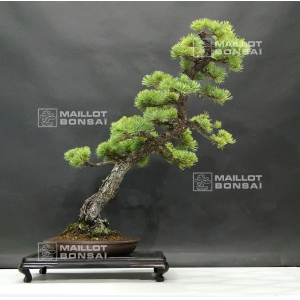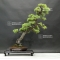##### The Japanese Bonsai specialist
Direct order Contact Help / Services Newsletter# Pinus pentaphylla 6070186

Pinus pentaphyllaref. : 9984

1.185,00

voluminous and/or heavy product extra shipping of 12,00

Available quantity : 1Order

###### Description

Height of the tree without its pot : 700 mm. Width of the branches : 500 mm. Japanese artisanal quality non-enamelled pottery of : 315 x 52 mm. Bunjin ji style. Diameter of the trunk’s base : 60 mm. Nebari : 120 mm. Bonsaï aged of more than 40 years old with short blue-toned needles. Good-looking ramifications without strong cutting wound or wire marks. This tree presents a superb formed bark. Well-conical trunk. Presence of jins divided on the trunk. The top of this bonsaï was recently formed, its cutting wound is currently healing but it doesn’t affect the bonsaï’s beauty. Superb shari on its forward face, very discreet, and a really bigger one on its back face. They both can be whitened with jin liquid according to your personal choice. Knotted roots with much character.

Photographed in june 2019. Comes from Hashimoto’s japanese nursery.

Shelf non-included.

#bonsai 5.6 #trunk 3.5 #with 3.4 #masterpieces 3.2 #pentaphylla 3.1 #japanese 2.8 #without 2.7 #cutting 2.7 #formed 2.6 #superb 2.6

Formule
(( ROUND((CHAR_LENGTH(b.article_nom)-CHAR_LENGTH(REPLACE(b.article_nom, 'bonsai', '')))/LENGTH('bonsai')) + ROUND((CHAR_LENGTH(b.article_description)-CHAR_LENGTH(REPLACE(b.article_description, 'bonsai', '')))/LENGTH('bonsai')) ) * 3.6) + (( ROUND((CHAR_LENGTH(b.article_nom)-CHAR_LENGTH(REPLACE(b.article_nom, 'trunk', '')))/LENGTH('trunk')) + ROUND((CHAR_LENGTH(b.article_description)-CHAR_LENGTH(REPLACE(b.article_description, 'trunk', '')))/LENGTH('trunk')) ) * 3.5) + (( ROUND((CHAR_LENGTH(b.article_nom)-CHAR_LENGTH(REPLACE(b.article_nom, 'with', '')))/LENGTH('with')) + ROUND((CHAR_LENGTH(b.article_description)-CHAR_LENGTH(REPLACE(b.article_description, 'with', '')))/LENGTH('with')) ) * 3.4) + (( ROUND((CHAR_LENGTH(b.article_nom)-CHAR_LENGTH(REPLACE(b.article_nom, 'pentaphylla', '')))/LENGTH('pentaphylla')) + ROUND((CHAR_LENGTH(b.article_description)-CHAR_LENGTH(REPLACE(b.article_description, 'pentaphylla', '')))/LENGTH('pentaphylla')) ) * 3.1) + (( ROUND((CHAR_LENGTH(b.article_nom)-CHAR_LENGTH(REPLACE(b.article_nom, 'japanese', '')))/LENGTH('japanese')) + ROUND((CHAR_LENGTH(b.article_description)-CHAR_LENGTH(REPLACE(b.article_description, 'japanese', '')))/LENGTH('japanese')) ) * 2.8) + (( ROUND((CHAR_LENGTH(b.article_nom)-CHAR_LENGTH(REPLACE(b.article_nom, 'without', '')))/LENGTH('without')) + ROUND((CHAR_LENGTH(b.article_description)-CHAR_LENGTH(REPLACE(b.article_description, 'without', '')))/LENGTH('without')) ) * 2.7) + (( ROUND((CHAR_LENGTH(b.article_nom)-CHAR_LENGTH(REPLACE(b.article_nom, 'cutting', '')))/LENGTH('cutting')) + ROUND((CHAR_LENGTH(b.article_description)-CHAR_LENGTH(REPLACE(b.article_description, 'cutting', '')))/LENGTH('cutting')) ) * 2.7) + (( ROUND((CHAR_LENGTH(b.article_nom)-CHAR_LENGTH(REPLACE(b.article_nom, 'formed', '')))/LENGTH('formed')) + ROUND((CHAR_LENGTH(b.article_description)-CHAR_LENGTH(REPLACE(b.article_description, 'formed', '')))/LENGTH('formed')) ) * 2.6) + (( ROUND((CHAR_LENGTH(b.article_nom)-CHAR_LENGTH(REPLACE(b.article_nom, 'superb', '')))/LENGTH('superb')) + ROUND((CHAR_LENGTH(b.article_description)-CHAR_LENGTH(REPLACE(b.article_description, 'superb', '')))/LENGTH('superb')) ) * 2.6) + (( ROUND((CHAR_LENGTH(b.article_nom)-CHAR_LENGTH(REPLACE(b.article_nom, 'wound', '')))/LENGTH('wound')) + ROUND((CHAR_LENGTH(b.article_description)-CHAR_LENGTH(REPLACE(b.article_description, 'wound', '')))/LENGTH('wound')) ) * 2.5)

## Secure payment## Delivery

Our logistic partners :